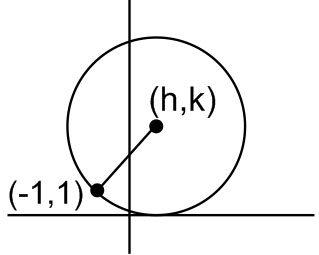#### Consider a family of circles which are passing through the point (–1, 1) and are tangent toaxis. Ifare the coordinate of the centre of the circles, then the set of values ofis given by the interval Option 1)Option 2)Option 3)Option 4)As we learnt in

General form of a circle -- wherein

centre =radius =Equation of circle with centeris:Radius of circle isWe get,Option 1)This option is incorrect.

Option 2)This option is incorrect.

Option 3)This option is incorrect.

Option 4)This option is correct.

#### divya.saini